## 2012年9月20日星期四

### Julia 初體驗の立法會選舉勝算 DIY

C++ 的發明人 Bjarne Stroustrup 將 C++ 形容為 "a general purpose programming language with a bias towards systems programming"。依此說法，Julia 大概就是 "a general purpose programming language with a bias towards scientific computing" 了。若不計 Julia 語法上對矩陣的直接支援，我想一般 programmers 應該不會將她聯想成 domain-specific language 吧。

Julia 今年一月才出 1.0 版本，算係有女初長成，距離亭亭玉立還有一段日子，現在仍有不少未成熟的地方，不過已經夠足我做練習用。前文提過，計算立法會選舉各競選名單的勝算，可用多項式分佈的常態逼近當成投票的分佈。利用蒙地卡羅模擬實驗，就可以計算出各名單的勝算。文友電鋸於選舉前已經做過類似的計算，此處只是當成我第一次的 Julia 編程練習。

$\mathbf{p}=\frac{1}{101} (4, 7, 5, 7, 1, 2, 16, 9, 3, 3, 8, 4, 1, 8, 1, 12)^\top,\ n=503.$
（由於 NOW 新聞台四捨五入，以上向量內各數字的總和為 101 而非 100。）我們的做法，是模擬多次投票實驗。每次實驗，均由 n=503 位選民，每人隨機投一張名單一票。投票的概率由 $\mathbf{p}$ 決定。換句話說，每一名選民都會有 $p_1=\frac4{101}$ 的機會投票予第一張名單、$p_2=\frac7{101}$ 的機會予第二張名單，餘此類推。當 503 人都投完票，就可以按比例代表制查出名單上各人是否當選，查核完畢，就完成了一次實驗。重覆同樣實驗許多次 ──  譬如 1,000,000 次，就完成了整個模擬過程。整個過程當中，若排在七號名單第二順位的余若薇當選了300,000 次，她當選的概率就估計為 300,000/1,000,000 = 0.3，其他人的當選概率也用同一方式估計。

n = 503 位選民每人按 $\mathbf{p}$ 的概率來投票，即是說各名單得票 $(X_1,\ldots,X_m)$ （今屆新界西有 m=16 張競選名單）的分佈為 $\textrm{Multinomial}(n, \mathbf{p})$。多項式分佈的常態逼近公式，可參考本網誌前文，當中用到的正交矩陣 Q 的構作方法，則見我另一篇網誌。總括來說，設

$\begin{eqnarray} v &=& \frac12 \left( \begin{bmatrix}0\\ \vdots\\0\\1\end{bmatrix} - \begin{bmatrix}\sqrt{p_1}\\ \vdots\\\sqrt{p_m}\end{bmatrix} \right),\\ M &=& \begin{bmatrix}\sqrt{\frac{p_1}n}\\ &\ddots\\&&\sqrt{\frac{p_m}n}\end{bmatrix} \left(I_m - 2\frac{vv^\top}{\|v\|^2}\right). \end{eqnarray}$若每次投票實驗，我們皆能夠生成 $m-1$ 個服從標準常態分佈的隨機數字 $Z_1, \ldots, Z_{m-1}$（重申，m 是競選名單數目，n 為投票人數），則每次實驗各名單的得票率可模擬為：

$\begin{bmatrix}\frac{X_1}n\\ \vdots\\ \frac{X_m}n\end{bmatrix}\approx \mathbf{p} + M\begin{bmatrix} Z_1\\ \vdots\\ Z_{m-1}\\ 0\end{bmatrix}.$

$\mathbf{f} = (f_1, \ldots, f_m)^\top = m\left(\frac{X_1}n, \ldots, \frac{X_m}n\right)^\top,$

1. 郭家麒 (1.0)
2. 譚耀宗 (1.0)
3. 李卓人 (0.999993)
4. 田北辰 (0.99991)
5. 李永達 (0.99954)
6. 梁耀忠 (0.999528)
7. 陳偉業 (0.996816)
8. 麥美娟 (0.996756)
9. 陳樹英 (0.918242)
10. 余若薇 (0.821678)
11. 陳恒鑌 (0.716208)
12. 梁志祥 (0.716037)
13. 陳一華 (0.338804)
14. 何君堯 (0.338146)
15. 龍瑞卿 (0.066209)
16. 曾健成 (0.065039)
17. 譚駿賢 (0.004338)
18. 麥業成 (0.002589)
19. 陳強 (0.002561)
20. 張慧晶 (0.000565)
... 等等。一百萬次實驗，在我的電腦上需時共廿九秒。至於何謂有「極高機會」當選，何謂「較高機會」、「機會均等」、「機會較低」，就見仁見智了。

## 2012年9月17日星期一

### Fate/Legco: 機器學習與選舉工程

\begin{align*} &\phantom{=}李永達與\ 9\ 位當選者的總得票率\\ &= \frac1{10} + p_1 + \ldots + p_9\\ &> \frac1{10} + \underbrace{\frac1{10} + \ldots + \frac1{10}}_{9 個} \quad（因為每個\ p_i\ 都大過 \frac1{10}）\\ &= 1. \end{align*}

$d_1(n)=\frac1{n+1}$ 的票，不管其他對手取得幾多票，此君亦鐵定當選，否則就會出現「部份候選人的得票率總和竟然大於 1」這件邏輯上不可能發生的事。上面這個 $d_1$，就是一般文章所講的 Droop quota，也就是保證排於名單首位的候選人取得一席所需的安全線

Droop quota 只是一條安全線，它是當選的充份條件 (sufficient condition) 而非必要條件 (necessary condition)。候選人越過它，則鐵定當選，但不越過也有可能當選。以今屆立法會選舉新界西的結果為例（下表，綠色當選，紅色落選），十六張名單爭奪 n=9 個議席，安全線為 $d_1(9) = \frac1{9+1} = 0.1$。從表中可見，九名當選者之中，只有郭家麒一人越過安全線（即是得票率 $\ge 0.1$）。

$\textrm{normalized proportion of votes}\ v' = \frac{v}{d_1(n)} =(n+1)v.$v' 愈接近 1，即代表該選舉名單愈接近安全線。搜集從 1998 年起四屆立法會的選舉數據，可將歷屆各參選名單的規範得票率與選舉結果圖列如下。（由於 $v'\ge1$ 就必然當選，故下圖並不包括 $v'\ge 1$ 的例子。）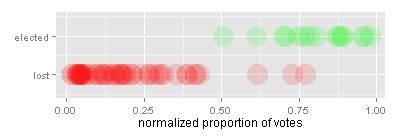圖一：1998-2008 年立法會選舉各候選名單的規範得票率圖二：用概率曲線稱合 1998-2008 年的規範得票率

$f(v') = \frac{1}{1+\exp(7.899974-12.664143v')}.$圖三：從九月七日民調所得的民主黨勝算曲線

1. （全攻型）務求全取兩席。
2. （防守型）確保一席，並伺機提高另一張名單的勝算，情況轉壞則棄車保帥。圖四：總得票率 0.14，全勝機會 0.5 或以上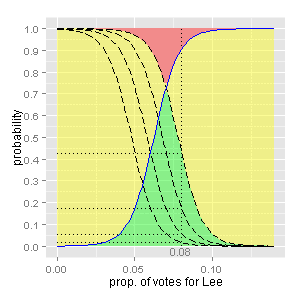圖五：民主黨的全勝機會，對總得票率十分敏感圖六：民主黨的勝算曲線 ($v_\max=0.13$)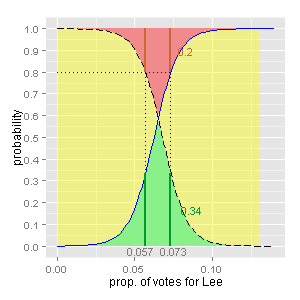圖七：民主黨的勝算曲線 ($v_\max=0.13$)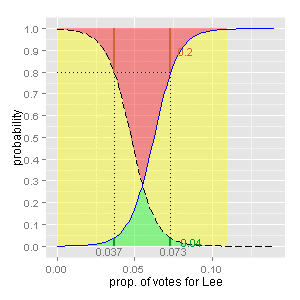圖八：民主黨的勝算曲線 ($v_\max=0.11$)

（至於誰是車，誰是帥，就很難說。我自己就比較希望棄李保陳，但這是另話。）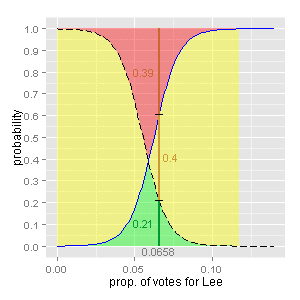圖九：事後孔明圖 ($v_\max=0.1177, v=0.0658$)
Fate/Legco

## 2012年9月15日星期六

### Normal approximation of multinomial distribution

How to simulate relative frequency outcomes of a multinomial experiment using normally distributed random numbers? The answer is surprisingly simple, but for some curious reason, it is seldom mentioned on the internet.

Let $\mathbf{X} = (X_1, \ldots, X_m) \stackrel{\textrm{i.i.d.}}{\sim} \textrm{Multinomial}(n, \mathbf{p})$, where $\mathbf{p}=(p_1,\ldots,p_m)$ is a probability vector whose entries sum to 1. In other words, we are talking about $n$ independent trials of a multinomial experiement, in which the probability of getting outcome $i\in\{1, 2, \ldots, m\}$ in each trial is $p_i$, and $X_i$ is the frequency count for outcome $i$ after all $n$ trials are completed. We have the following:

Theorem. Let $u = (\sqrt{p_1},\ldots,\sqrt{p_m})$ and $Q$ be a real orthogonal matrix whose last column is $u$. Suppose $Z_1, \ldots, Z_{m-1}$ are $m-1$ i.i.d. standard normal random variables. Then
$\mathbf{X}^\ast = \left( \frac{X_i-np_i}{\sqrt{np_i}} \right)_{1\le i\le m} \stackrel{d}{\longrightarrow}\quad Q \begin{bmatrix} Z_1\\ \vdots\\ Z_{m-1}\\ 0\end{bmatrix}$as $n\rightarrow\infty$.

Proof. See sec. 11.1 of Hans-Otto Georgii (2007), Stochastics: Introduction to Probability and Statistics, Walter de Gruyter, Berlin.

*****************************
Note that the denominator of the $i$-th entry of $\mathbf{X}^\ast$ in the above theorem is $\sqrt{np_i}$, not the usual $\sqrt{np_i(1-p_i)}$ we see in the normal approximation formula to binomial distribution. We will immediately see why the binomial case is just a special case of the above general formula. Let $m=2$ and write $\mathbf{p}=(p,q)^\top$. Take $Q$ as $\begin{pmatrix}\sqrt{q} & \sqrt{p}\\ -\sqrt{p} & \sqrt{q}\end{pmatrix}$. So, the above theorem says that
$\begin{bmatrix} \frac{X_1-np}{\sqrt{np}}\\ \frac{X_2-nq}{\sqrt{nq}}\end{bmatrix}\stackrel{d}{\longrightarrow} \begin{pmatrix}\sqrt{q} & \sqrt{p}\\ -\sqrt{p} & \sqrt{q}\end{pmatrix} \begin{bmatrix} z\\ 0\end{bmatrix}= \begin{bmatrix} \sqrt{q}\,Z\\ -\sqrt{p}\,Z\end{bmatrix}.$Hence, by Continuous Mapping Theorem, we can divide both sides entrywise by $(\sqrt{q}, -\sqrt{p})^\top$ and get
$\begin{bmatrix} \frac{X_1-np}{\sqrt{npq}}\\ \frac{nq-X_2}{\sqrt{npq}}\end{bmatrix} \stackrel{d}{\longrightarrow} \begin{bmatrix} Z\\ Z\end{bmatrix}.$
Since $X_1+X_2= n$, we have $nq-X_2=X_1-np$. Hence the above convergence reduces to the familiar normal approximation formula to binomial distribution.

The theorem requires the use of a real orthogonal matrix $Q$ whose last column is $u$. How to construct such a matrix? See my previous blog entry.

By the theorem, the covariance matrix of the approximating distribution is given by $Q\ \mathrm{diag}(1,\ldots,1,0)\ Q^\top = I-uu^\top$. This matrix is, of course, degenerate because the $X_i$'s are not independent of each other (as $\sum_{i=1}^m X_i=n$).

## 2012年9月13日星期四

### 大佬

Roy：如果你夠膽同我講，以後唔認我做大佬？我等你。

（忽然，㩒釘華一手將牛肉刀劈進 Roy 頸中。）
（Roy 倒地，一命嗚呼。）

## 2012年9月9日星期日

### A handy completion of orthogonal matrix from a column vector

Given a unit vector $u=(u_1,u_2,\ldots,u_n)^\top$, we seek to construct an orthogonal matrix $Q$ whose first column is $u$.

If $u=e_1=(1,0,\ldots,0)^\top$, clearly we can pick $Q=I$. Suppose $u\ne e_1$. Then the Householder reflection $Q = I - 2vv^\top$ will do, where
$v = \frac{u-e_1}{\|u-e_1\|}.$
In particular, if $u=\frac{1}{\sqrt{n}}e=(\frac{1}{\sqrt{n}},\frac{1}{\sqrt{n}},\ldots,\frac{1}{\sqrt{n}})^\top$, we may choose
$Q=\pmatrix{a&a&\cdots&\cdots&a\\ a&b+1&b&\cdots&b\\ \vdots&b&b+1&\ddots&\vdots\\ \vdots&\vdots&\ddots&\ddots&b\\ a&b&\cdots&b&b+1}$
where $a=\frac{1}{\sqrt{n}}$ and $b=\frac{-1}{n-\sqrt{n}}$.

One question remains. When $u\approx e_1$, how stable is this construction?